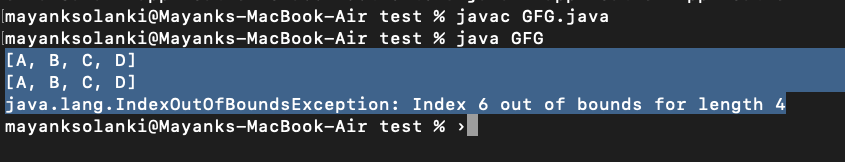# How to Replace a Element in Java ArrayList?

To replace an element in Java ArrayList, set() method of java.util. An ArrayList class can be used. The set() method takes two parameters-the indexes of the element which has to be replaced and the new element. The index of an ArrayList is zero-based. So, to replace the first element, 0 should be the index passed as a parameter.

Declaration:

`public Object set(int index, Object element)`

Return Value: The element which is at the specified index

Exception Throws: IndexOutOfBoundsException
This occurs when the index is out of range.

`index < 0 or index >= size()`

Implementation:

Here we will be proposing out 2 examples wherein one of them we will be setting the index within bound and in the other, we will be setting the index out of bounds.

Example 1: Where Index is Within Bound

## Java

 `// Java program to demonstrate set() Method of ArrayList` `// Where Index is Within Bound `   `// Importing required classes` `import` `java.io.*;` `import` `java.util.*;`   `// Main class` `class` `GFG {`   `  ``// Main driver method` `  ``public` `static` `void` `main(String[] args) {`   `    ``// Try block to check for exceptions` `    ``try` `{`   `      ``// Creating an object of Arraylist class` `      ``ArrayList list = ``new` `ArrayList<>();`     `      ``// Adding elements to the List` `      ``// using add() method`   `      ``// Custom input elements` `      ``list.add(``"A"``);` `      ``list.add(``"B"``);` `      ``list.add(``"C"``);` `      ``list.add(``"D"``);`   `      ``// Print all the elements added in the above object` `      ``System.out.println(list);`   `      ``// 2 is the index of the element "C".` `      ``//"C" will be replaced by "E"` `      ``list.set(``2``, ``"E"``);`   `      ``// Printing the newly updated List` `      ``System.out.println(list);`   `    ``}`   `    ``// Catch block to handle the exceptions` `    ``catch` `(Exception e) {`   `      ``// Display the exception on the console` `      ``System.out.println(e);` `    ``}` `  ``}` `}`

Output

```[A, B, C, D]
[A, B, E, D]
```

Example 2: Where Index is Out of Bound

## Java

 `// Java program to demonstrate set() Method of ArrayList ` `// Where Index is Out of Bound`   `// Importing required classes` `import` `java.io.*;` `import` `java.util.*;`   `// Main class` `class` `GFG {`   `  ``// Main driver method` `  ``public` `static` `void` `main(String[] args) {`   `    ``// Try block to check for exceptions` `    ``try` `{`   `      ``// Creating an object of Arraylist class` `      ``ArrayList list = ``new` `ArrayList<>();`     `      ``// Adding elements to the List` `      ``// using add() method`   `      ``// Custom input elements` `      ``list.add(``"A"``);` `      ``list.add(``"B"``);å` `      ``list.add(``"C"``);` `      ``list.add(``"D"``);`   `      ``// Print all the elements added in the above object` `      ``System.out.println(list);`   `      `  `      ``// Settijg the element at the 6 th index which` `      ``// does not exist in our input list object ` `      ``list.set(``6``);` `  `  `      ``// Printing the newly updated List` `      ``System.out.println(list);` `    ``}`   `    ``// Catch block to handle the exceptions` `    ``catch` `(Exception e) {`   `      ``// Display the exception on the console` `      ``System.out.println(e);` `    ``}` `  ``}` `}`

Output:Whether you're preparing for your first job interview or aiming to upskill in this ever-evolving tech landscape, GeeksforGeeks Courses are your key to success. We provide top-quality content at affordable prices, all geared towards accelerating your growth in a time-bound manner. Join the millions we've already empowered, and we're here to do the same for you. Don't miss out - check it out now!

Previous
Next